# Bridgeless Boost Rectifier for Low Voltage Energy Harvesting Applications

Bridgeless Boost Rectifier for Low Voltage Energy Harvesting Applications : A bridgeless boost rectifier for low voltage energy harvesting, is a single stage ac to dc power electronic converter that is used for low voltage but high current power electronic applications. This converter has made by combing the buck and boost converter in a single circuit for converting the positive and negative half cycle of ac voltages into dc voltages. Only one inductor and capacitor has been used for reducing the size of this power electronic converter. Here we will be told the user, how we can make this bridgeless boost rectifier for low voltage energy harvesting application power electronic converter with the help of MATLAB Simulink model as well as we also told the user how we can make a new model on MATLAB. For making a new circuit on MATLAB first we must to make a new model and for making a new model, first we will open the MATLAB and then click on MATLAB Simulink library. By clicking on MATLAB Simulink library, a new page would be opened, that is known as Simulink library browser shown in figure 1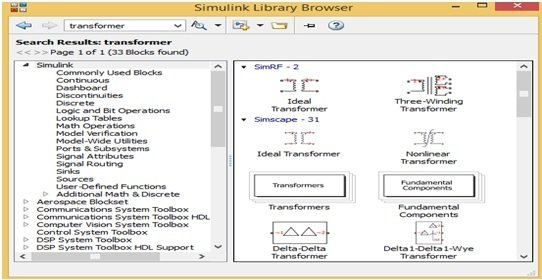Figure 1 MATLAB Simulink Library Browser

This MATLAB Simulink library browser contains all the user related components blocks and for searching any component block just writ the specific right name of that component at search Manu bar then this library browser opens the all the related blocks of that component. When the user wants to make a new circuit, then first he will click on new model option, which is exist on Manu bar of this page. When he will click on new model then a new page would be opened shown in figure 2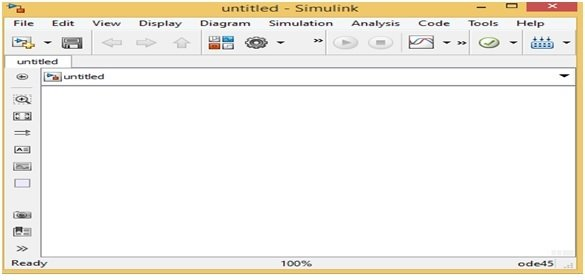Figure 2 Figure 2 MATLAB Simulink New Model

In this new model page, the user can easily make any new circuit and for making new circuit the user first will find the components blocks. Suppose, the user wants to make the power electronics circuit then first he will find the power electronic blocks by writing the word power electronic on search Manu bar. When he will write the word power electronic on search Manu bar then a new page would be opened shown in figure 3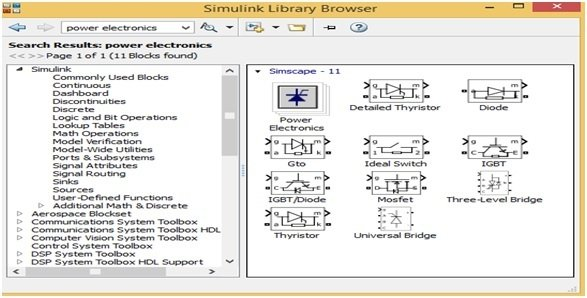Figure 3 Power Electronics Components Blocks

This page has all the power electronic components block as per the requirement of user. Now the user can move these components blocks on a new model page either by dragging or by right clicking on every block and then click on option at add block to a new model. Then the block will automatically shift to a new model page. Similarly, the user can easily search and drag all these other components blocks such as PWM generator, Oscilloscope, inductor and capacitor etc. By following all these above instructions, the user can easily make any new circuit or new model in MATLAB Simulink. Similarly, for making the bridgeless boost rectifier for low voltage energy harvesting application power electronic converter the user will fist make the circuit according to below block diagram of bridgeless boost rectifier for low voltage energy harvesting application shown in figure 4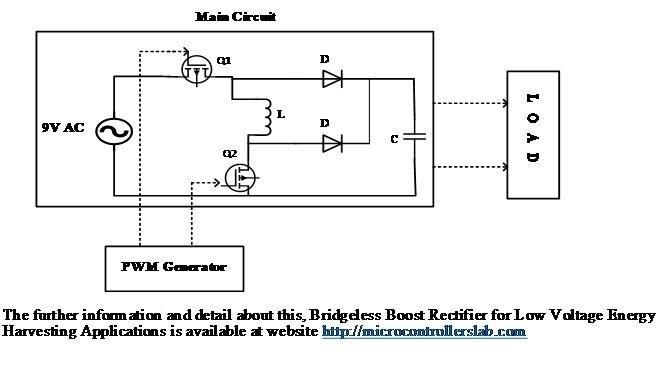Figure 4 Block Diagram of Bridgeless Boost Rectifier for Low Voltage Energy Harvesting Applications

This bridgeless boost rectifier for low voltage energy harvesting application power electronic converter works in four modes of operation. In mode one both switches Q1 and Q2 are on simultaneously, when both are on simultaneously then inductor would be charged and both diode would be reversed biased. In mode 2 the switch Q1 would be on, Q2 would be off and diode D2 would be forward biased and inductor would be discharged and provide the voltage to output load. This process would be occurred in positive half cycle of ac voltage. Similarly, in negative half cycle the same process would be occurred but in second mode Q2 would be on and Q1 would be off. The PWM is given by switches Q1 and Q2 through function generator.

### simulation and simulink circuit diagram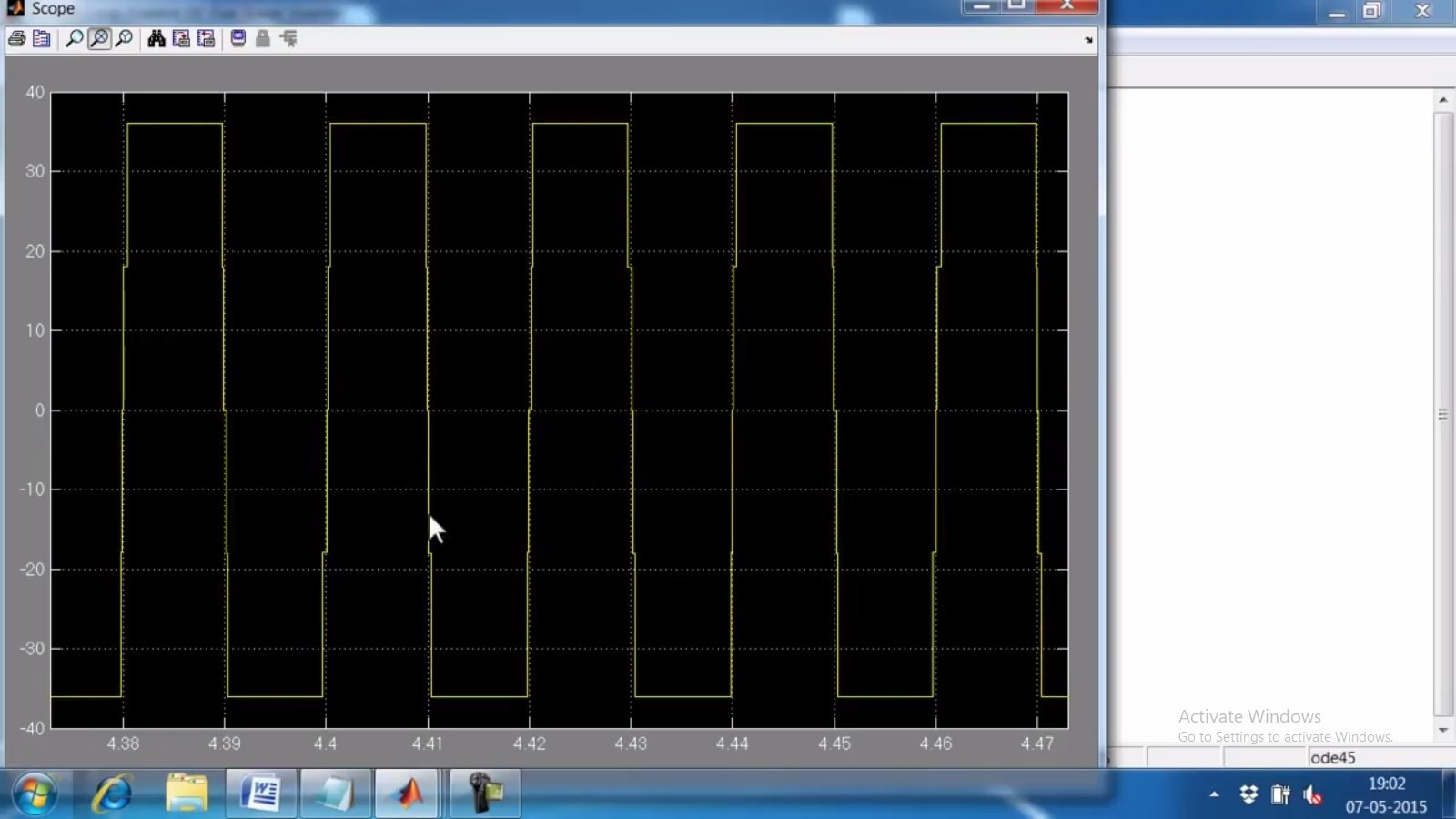### 1 thought on “Bridgeless Boost Rectifier for Low Voltage Energy Harvesting Applications”

1. Hola Bilal
Fabricamos rectificadores de alta frecuencia hasta 2500w, con entrada monofasica y trifasica en modo de tres módulos monofasicos
Usamos puente H con mosfet
Driveamos con 2110
Nos faltaría contar con un PFC que permita controlar distorsión de corrinete y armonicos de 3 / 5 y 7° armónicas
Podríamos ver si tenes algo o bien me informas sobre el tema
Abrazo
Oscar